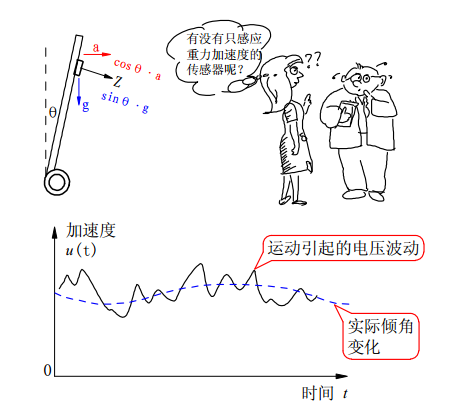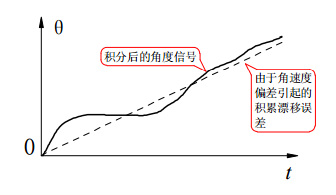互补滤波器//———————我是华丽丽的分根线———————//

• 低通滤波器
• 高通滤波器
• 带通滤波器

（一阶）互补滤波核心代码非常简洁：

angle = (0.98)*(angle + gyro * dt) + (0.02)*(x_acc);

积分器

position += speed*dt;

angle += gyro*dt;

低通滤波器

angle = (0.98)*angle_last + (0.02)*x_acc;

（一阶）互补滤波器（二阶）互补滤波器

// newAngle 为加速度传感器经过atan2()转化后的角度值
// newRate 为陀螺仪角度值
// looptime 为计算周期
float Complementary2(float newAngle, float newRate, intlooptime)
{
float k = 10;
float dtc2 = float(looptime) / 1000.0;
x1 = (newAngle -   x_angle2C) * k * k;
y1 = dtc2 * x1 + y1;
x2 = y1 + (newAngle -   x_angle2C) * 2 * k + newRate;
x_angle2C = dtc2 * x2 + x_angle2C;
return x_angle2C;
}